# Create Mesh

All visible objects in 3D scene are meshes. Different meshes has different shapes. In previous sections, we once created face meshes such as rectangle planes, cubes, spheres, we also created line geometries such as coordinate meshes. In this section, we'll discuss how to create meshes with different shapes.

## Primitive Meshes

Primitive meshes includes rectangle plane, cubes, spheres, cylinders, cones. We created planes and cubes before, now let's see how to create spheres, cylinders and cones.

1. Use `MeshBuilder.createSphere(scene, radius, widthSegs, heightSegs)` to create sphere.
2. Use `MeshBuilder.createCylinder(scene, radius, height, segs)` to create cylinder.
3. Use `MeshBuilder.createCone(scene, radius, height, segs)` to create cone.

When creating a mesh, you need to specify the scene. You need to specify the radius when creating a sphere; and you need to specify radius and height when creating a cylinder or a cone.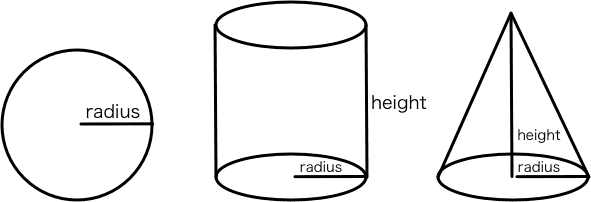These meshes' surface is curved, G3D use line strip to simulate curve line. For example, a circle could be roughly simulated using 6 lines, or nicely simulated using 128 lines. The `segs` argument is used to specify how many lines should be used to simulate a circle. The larger the number is, the nicer G3D simulates (and cost more, of course).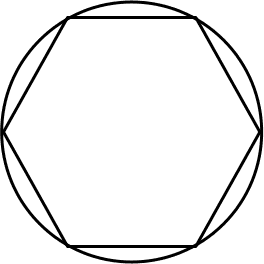This example demonstrated how to create sphere, cylinder and cones, with different `segs` arguments.

We created three cylinders, three spheres and three cones, with `segs` 6, 16 and 128. It's obvious that segs deeply affect how nice the curved face is simulated.

## Meshes with Custom Shapes

Except for these pre-defined shaped meshes, we can alse mannually create vertices and make up our own custom shaped meshes. It's a little bit tricky, so let's begin with a simple issue: How to create custom shaped line mesh. (Spheres, cubes and so on are face meshes, but the coordinate mesh we created before is line mesh).

### Line Mesh

Assume that we want to create a line strip in X-Y plane, passing through (0, 0, 0), (1, 1, 0), (1, 0, 0), (2, 1, 0), (2, 0, 0) and so on, as the following image shows: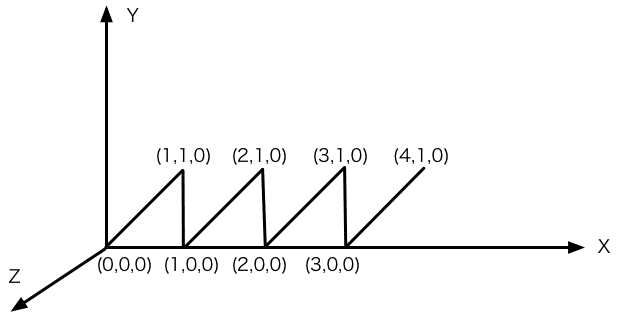Let's see the demo:

First, create two arrays `vertices` and `indices`:

``````const vertices = [];
const indices = [];
for(let i=0; i<4; i++){
vertices.push(0+i, 0, 0, 1+i, 1, 0);
if(i!==0){
indices.push(i*2-1, i*2);
}
indices.push(i*2, i*2+1);
}

// vertices: [0,0,0, 1,1,0, 1,0,0, 2,1,0, 2,0,0, 3,1,0, ...]
//            0      1      2      3      4      5
// indices:  [0,1, 1,2, 2,3, 3,4, 4,5, ...]``````

The array `vertices` container all vertice positions along the line strip, each three values for a vertices. The line containers 8 vertices, so the length of `vertices` is 24.

The array `indices` defines how the vertices in `vertices` composite a line strip. Each value in `indices` is an index for `vertices` array, corresponding with a vertice. In the example, `indices` is [0,1,1,2,...]. The first two value 0 and 1 means: The first line, start with the 0th vertice in `vertices` which is (0, 0, 0), ends with the 1st vertice which is (1, 1, 0); the following two value 1 and 2 means: the second line, start with 1st vertice which is (1, 1, 0) and ends with the 2nd vertice which is (1, 0, 0); and so on.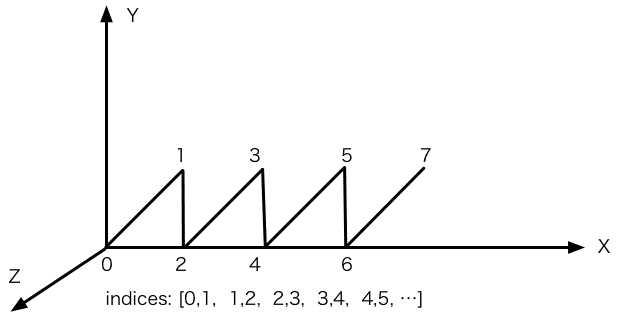Then we can create a `LineMesh` object, and create a `LineGeometry`, passing `vertices` and `indices` in. Notice that we put `indices` in a `default` property, this is because a line mesh may contain multiple line strips, the first one's name is `default` by default.

``````const lines = new G3D.LineMesh(scene);
lines.geometry = new G3D.LineGeometry({
vertices,
indices: {
default: indices
}
})``````

So we finished creating a custom shaped line mesh.

### Face Mesh

Creating face mesh is a little complecated. Assum that we want to create a face mesh composited of two rectangle planes ABCD and EFGH: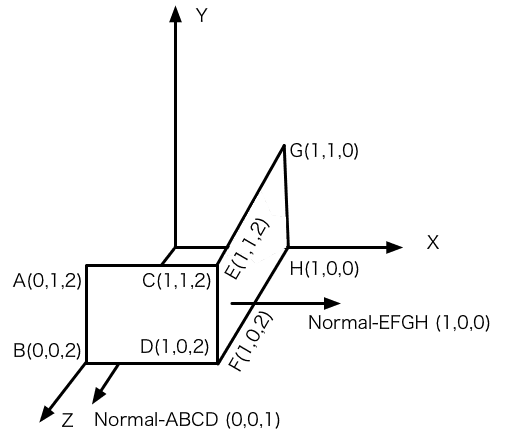See the example:

Except for `vertices` and `indices`, we need to create `uvs` and `normals`.

``````const vertices = [
0,1,2, // A
0,0,2, // B
1,1,2, // C
1,0,2, // D
1,1,2, // E
1,0,2, // F
1,1,0, // G
1,0,0  // H
];
const normals = [];
const uvs = [];
for(let i=0; i<8; i++){
if(i<=3){
normals.push(0,0,1);
}else{
normals.push(1,0,0);
}
uvs.push(0,0);
}``````

The array `vertices` container 8 points, which is the similar to when we created line mesh before.

Normal is the vector perpendicular to the face. For line meshes, normals makes no sense, but for face meshes, normals participates in computing the light and the final render color, it's essantial. In our example, for the first four vertices, normal is (0, 0, 1), and for the 4 vertices left, normal is (1, 0, 0).

You may notice that the mesh has only 6 vertices literally, cause point C and point E is the same point, as well as D and F. C and E has the same position, but the normal is different, so we have to split it into two points.

UV is similar to normals, it's data for each vertices, but UV only need two values for one vertice. UV is related to the texture and material, as we have not involved in these issues, we can just specify (0, 0) for each vertice.

At last we created the vertice index array `indices`. For line geometry, the rule is "each two indices make up a line segment", and here for face geometry, the rule is "each three indices make up a triangle". Face meshes are composited with triangles, so rectangle plane should be split into two triangles. Here in the example, `indices` demonstrate 4 triangles, they are triangle ABC and BDC (which composite rectangle ABCD), and triangle EFH and HGE (which composite rectangle EFGH).

``````const indices = [
0,1,2, // triangle ABC
1,3,2, // triangle BDC
4,5,7, // triangle EFH
7,6,4  // triangle HGE
]``````

At last, create a `Mesh`. We used `LineMeshed` and `LineGeometry` before, here we need `Mesh` and `Geometry`. For the same reason, `indices` is in the `default` property.

``````const mesh = new G3D.Mesh(scene);
mesh.geometry = new G3D.Geometry({
vertices,
normals,
uvs,
indices: {
default: indices
}
});``````

Please drag your mouse in the right rendering canvas, to rotate the camera to the backside of our mesh (or you can edit code to change the camera, `camera.alpha = 200`). You find that when camera looks at the mesh from back side, the mesh is gone! (This won't happen for the mesh you created from `MeshBuilder.createPlane()`).

This is because, mostly face mesh is closed, you are not supposed to see the back side of a plane. For better performance, we'll not render the back side. In G3D, `Geometry` has a 'facing', when creating `Geometry`, you can specify a `facing` property as `Geometry.FACING.FRONT` (the default value)，`Geometry.FACING.BACK` or `Geometry.FACING.BOTH`.

So if we want the mesh to be seen from both side, then specify `BOTH` as following code shows:

``````mesh.geometry = new G3D.Geometry({
...
facing: G3D.Geometry.FACING.BOTH
})``````

How to recognize the front side and back side? Here we use the Right Hand Rule again. Use your right hand, make the four fingers rotate along the triangle vertices in order (A->B->C, eg), then the thumb is pointing at the front side.

## Summary

In this section we learned: 1) How to create pre-defined shaped meshes using `MeshBuilder`. 2) How to create custom shaped meshes. Hope the artical is helpful.